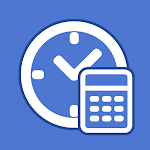ID: com.kouji1981.time

• Author:

• Version:

Varies with device

• Update on:

## The description of Time Calculator

It is an application that can easily calculate the elapsed time from the time and the time from the elapsed time.
You can also display the time between the start date and time and the end date and time in terms of hours, minutes, seconds, etc.

・Calculate from start time and end time
Enter the start time and end time to calculate the elapsed time.
Elapsed time can be checked in terms of hours, minutes, and seconds, and the current time can be easily entered.

・Calculated from start time + elapsed time
Calculate the end time with the start time and elapsed time.
You can also ask for the time before the elapsed time.

・Calculate from the start date and time and the end date and time
Enter a start date and time and an end date and time to calculate the elapsed time.
Elapsed time can also be checked in terms of hours, minutes, and seconds.

・Calculated from start date and time + elapsed time
Enter the start date and time and elapsed time to calculate the end date and time.

・Time conversion
The entered time is displayed in day/hour/minute/second, converted to days, converted to hours, converted to minutes, or converted to seconds.
You can also convert 1000 seconds into minutes and seconds, or convert hours into days.